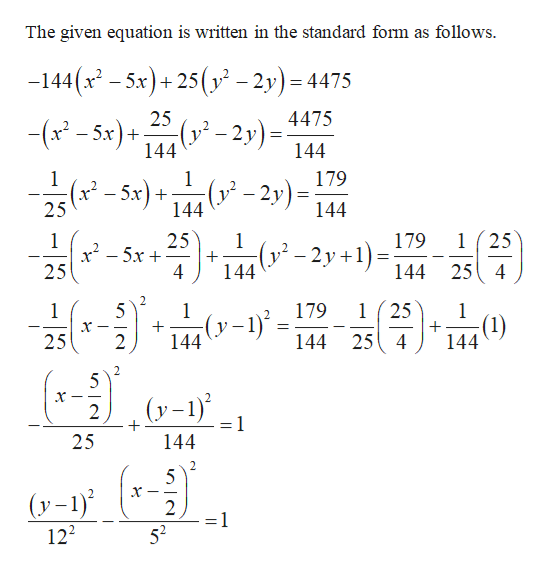# For Exercise,a. Write the equation of the hyperbola in standard form.b. Identify the center, vertices, and foci.−144x2 + 25y2 + 720x − 50y − 4475 = 0

Question
1 views

For Exercise,

a. Write the equation of the hyperbola in standard form.

b. Identify the center, vertices, and foci.

−144x2 + 25y2 + 720x − 50y − 4475 = 0

check_circle

Step 1

The given hyperbola equation is,

Step 2help_outlineImage TranscriptioncloseThe given equation is written in the standard form as follows. -144(x – 5x)+ 25(y² – 2y) = 4475 25 4475 (v² – 2y)=: -(x - 5x) + 144 144 179 (² - 5x)- (1² – 2y) = 144 25 144 1 (25 179 25 x² – 5x + 4 (² – 2y +1)= 25 4 25 144 144 2. 1 ( 25 179 (y-1)° = 144 (y–1) 144 (1) 144 х 25 4 25 х 25 144 2. (y-1) 122 52 fullscreen

### Want to see the full answer?

See Solution

#### Want to see this answer and more?

Solutions are written by subject experts who are available 24/7. Questions are typically answered within 1 hour.*

See Solution
*Response times may vary by subject and question.
Tagged in

### Other